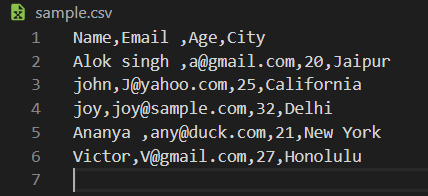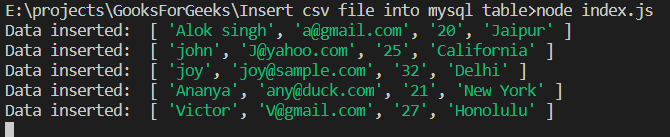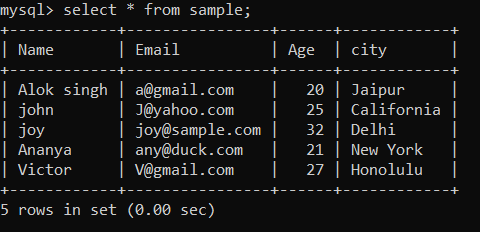# How to import data from .CSV file into MySQL table using Node.js ?

• Difficulty Level : Basic
• Last Updated : 19 Jan, 2022

What is .CSV file?
The .CSV (Comma Separated Values) files are plain text files that contains a list of data separated by comma(,). It is a format best used for tabular data, row, and columns, exactly like a spreadsheet, just the difference is that the file is in the form of plain text. The idea of a CSV file is to perform export and import complex data from one application to another.

Note: Here, we are using sample.csv to demonstrate our program and procedure. In sample.csv we have stored the user’s Name, Age, Email, and City.Data in sample.csv

Import CSV into MySQL using Node.js:

1. Create a node.js project using “npm init” and save your .csv file in the same directory.
2. Install two packages “mysql” and “csvtojson” using the following command:
`npm i mysql csvtojson`

mysql driver: This is a node.js driver for mysql. It is written in JavaScript, that does not require compiling. We are using it to establish connection with our MYSQL database and perform queries.
csvtojson: Its a csv parser to convert csv to json or column arrays.

3. Now just create a file like index.js and write down the following code:

 `// Importing mysql and csvtojson packages``// Requiring module``const csvtojson = require(``'csvtojson'``);``const mysql = require(``"mysql"``);`` ` `// Database credentials``const hostname = ``"localhost"``,``    ``username = ``"root"``,``    ``password = ``"root"``,``    ``databsename = ``"csvtomysql"`` ` ` ` `// Establish connection to the database``let con = mysql.createConnection({``    ``host: hostname,``    ``user: username,``    ``password: password,``    ``database: databsename,``});`` ` `con.connect((err) => {``    ``if` `(err) ``return` `console.error(``            ``'error: '` `+ err.message);`` ` `    ``con.query(``"DROP TABLE sample"``, ``        ``(err, drop) => {`` ` `        ``// Query to create table "sample"``        ``var` `createStatament = ``        ``"CREATE TABLE sample(Name char(50), "` `+``        ``"Email char(50), Age int, city char(30))"`` ` `        ``// Creating table "sample"``        ``con.query(createStatament, (err, drop) => {``            ``if` `(err)``                ``console.log(``"ERROR: "``, err);``        ``});``    ``});``});`` ` `// CSV file name``const fileName = ``"sample.csv"``;`` ` `csvtojson().fromFile(fileName).then(source => {`` ` `    ``// Fetching the data from each row ``    ``// and inserting to the table "sample"``    ``for` `(``var` `i = 0; i < source.length; i++) {``        ``var` `Name = source[i][``"Name"``],``            ``Email = source[i][``"Email"``],``            ``Age = source[i][``"Age"``],``            ``City = source[i][``"City"``]`` ` `        ``var` `insertStatement = ``        ```INSERT INTO sample values(?, ?, ?, ?)`;``        ``var` `items = [Name, Email, Age, City];`` ` `        ``// Inserting data of current row``        ``// into database``        ``con.query(insertStatement, items, ``            ``(err, results, fields) => {``            ``if` `(err) {``                ``console.log(``    ``"Unable to insert item at row "``, i + 1);``                ``return` `console.log(err);``            ``}``        ``});``    ``}``    ``console.log(``"All items stored into database successfully"``);``});`

Run index.js file using the following command:

`node index.js`

### Output:Output of the program in consoleSample table in MYSQL database

Explanation of code:

• At the first two lines of code we Import  mysql and csvtojson.
```const csvtojson = require('csvtojson');
const mysql = require("mysql")```
• Line 10 – 23 : We created a connection to our database.
```hostname = "localhost",
databsename = "csvtomysql" // Database we are connecting to```
• Line 23 – 38: We have connected to our database “csvtomysql” and created table named “sample” with desired fields according to our sample.csv file.
• Line 42 – 64 : We fetched sample.csv located in current directory and converted all the data to JSON.
• At line 43 all data in sample.csv is converted to JSON and stored in variable “source”
• Then we loop through each each row and extracted Name, Email, Age and City value from that row.
• At line 53, we created a array of values in Name, Email, Age and City i.e. the column data of ith row.
• Then we inserted that data into table using  query “INSERT INTO sample values(Name, Email, Age, City)”
• At line 62 we are showing the inserted data to console.

So, this way we can import any data from a .csv file to our MYSQL database.

My Personal Notes arrow_drop_up